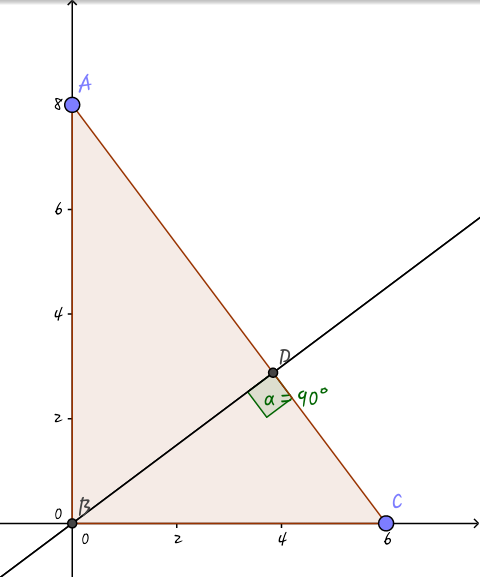# Think Geometry!

Geometry Level 3A right $\Delta$ $ABC$ is drawn on the $x-y$ plane as shown in the figure above.

If $AB$ is $8$ units and $BC$ is 6 units, and the value of $BD + CD$ is $k$, then enter the value of $\lfloor 100k \rfloor$.

×